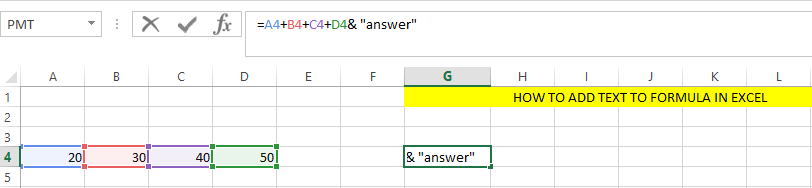#### How to add text to formula?

You may notice some problems when working with data. One of them is creating cells that contain different types of data.

## Using an ampersand

Here are the steps to add text to formula in Microsoft Excel.

Suppose I want to add values in Cell A4, B4, C4 and D4 and display the result in cell E4 along with a text message.

I will enter a value in Cell A4, B4, C4 and D4 I will click on cell G4 and then write =A4+B4+C4+D4 & “answer” in the formula bar and press enter.The result will be displayed as text in the cell where the formula is entered.

Note: any text written between ” ” will be displayed.

Important: Remember to add “&” (ampersand)

## Using the CONCAT() function

The CONCAT() function is a more powerful than the & (ampersand) operator for adding text to formulas. It allows you to combine multiple text strings and formulas into a single string.

The following formula uses the CONCAT() function to combine the text strings “Hello” and “World” into a single string:

`=CONCAT("Hello", " ", "World")`

This formula will return the string “Hello World”.

You can also use the CONCAT() function to combine text strings and formulas into a single string. For example, the following formula uses the CONCAT() function to combine the text string “The total sales for the day are” with the formula SUM(A1:A10) to create a single string that displays the total sales for the day:

`=CONCAT("The total sales for the day are ", SUM(A1:A10))`

This formula will return a string such as “The total sales for the day are 1000”.

`=TEXT(1000, "\$#,##0.00")`